# help turning input into user input in arcgis

1418
14
11-10-2017 12:28 PMNew Contributor II

velocity = float(input("Input asteroid velocity: "))
mass = float(input("Input asteroid mass: "))
ice = float(1.8)
iron = float(7.3)
rock = float(2.3)
comp = input("input 1 for ice, 2 for iron, or 3 for rock: ")
grav = float(0.16667)
const = float(0.007)
colrt = float(1.3)
soil = float(2.65)
power = (int(1)/float(3.4))
vel = (velocity * 1000)
kilotons = (4184000000000)
if comp == 1:
comp = ice
elif comp == 2:
comp = iron
elif comp == 3:
comp = rock
kinetic = (0.5 * mass * (vel * vel))
energy = (kinetic * kilotons)
part1 = (const * colrt * grav)
part2 = (comp / soil)
part3 = (energy * part2)
part4 = (part3 ** power)
crater = (part1 * part4)
final = (crater / int(1000))
print(final)

sorry for the length but i'm trying to take the velocity, mass, and comp and turn them into user input parameters that will be run through the equation and then have it spit out a diameter

my problem is trying to set up the user input part to work with my script. i eventually want to take the script add it to model builder and do more with the output of this script.

any help would be greatly accepted

1 Solution

Accepted SolutionsbyEsri Esteemed Contributor

It would have been good to read the help since it mentions exactly what you should do, but ok here goes a more compact explanation.

You can create a new toolbox or use an existing one and add a script: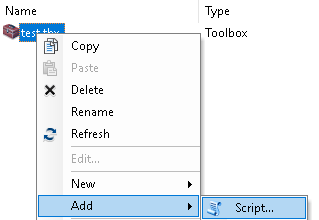You will have to add the existing Python script (see further down below):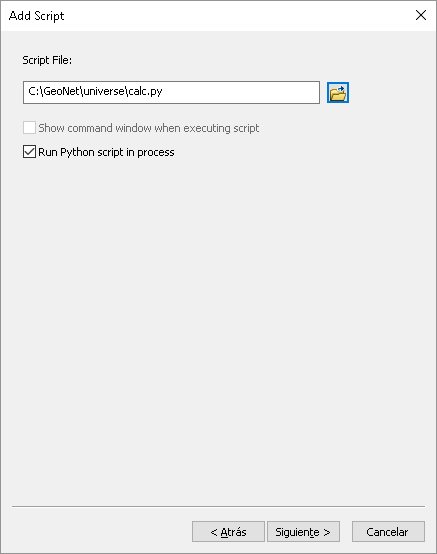This is what you configure as parameters: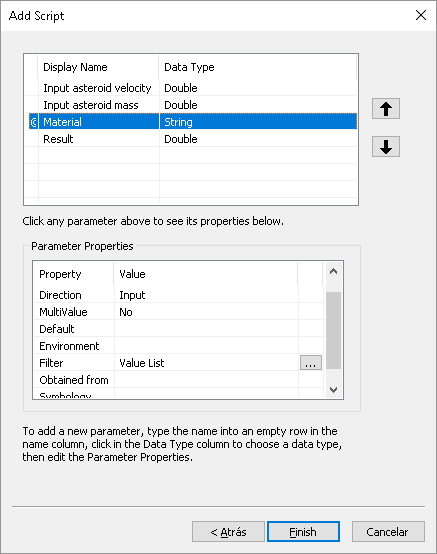In the lower part the Filter "Value List" will contain the list of materials: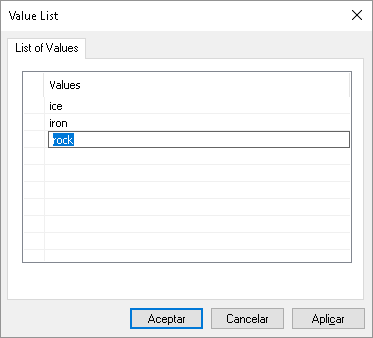The 4th parameters is the output and should have that "direction":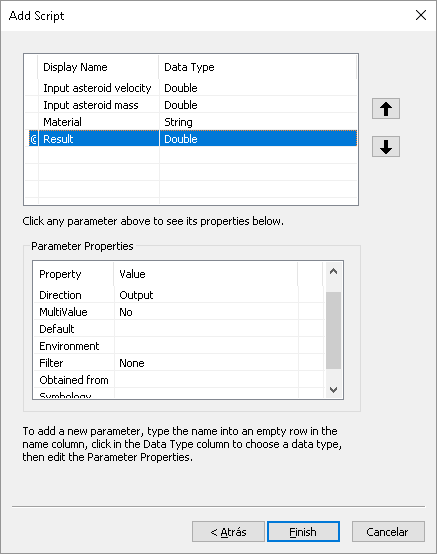When you execute the tool you can enter all the parameters:And the result will be: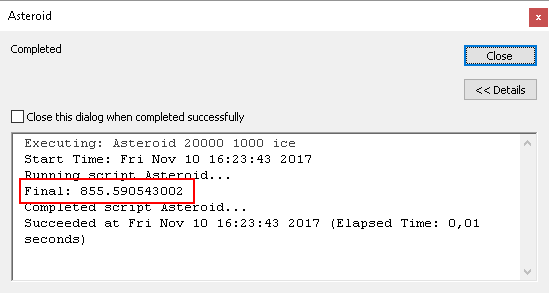When you load the tool in modelbuilder (simply drag and drop) you will see that you can use it there: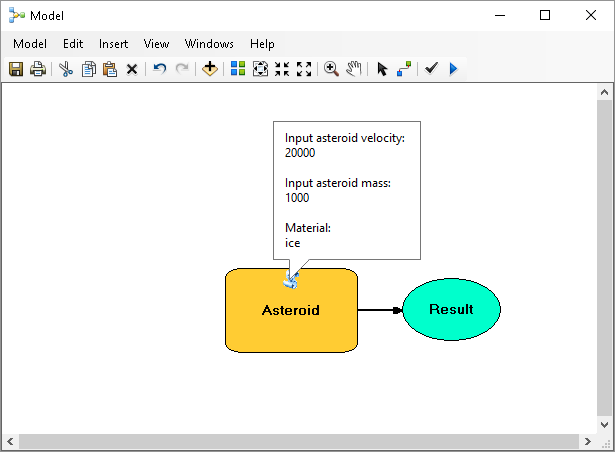Python script:

``````def main():
import arcpy

velocity = arcpy.GetParameter(0)
mass = arcpy.GetParameter(1)
component = arcpy.GetParameterAsText(2)

dct_mat = {'ice': 1.8, 'iron': 7.3, 'rock': 2.3}
comp = dct_mat[component]
grav = 0.16667
const = 0.007
colrt = 1.3
soil = 2.65
power = 1.0/3.4
vel = velocity * 1000.0
kilotons = 4184000000000.0

kinetic = (0.5 * mass * vel**2)
energy = (kinetic * kilotons)
part1 = (const * colrt * grav)
part2 = (comp / soil)
part3 = (energy * part2)
part4 = (part3**power)
crater = (part1 * part4)
final = (crater / int(1000))

arcpy.SetParameter(3, final)

if __name__ == '__main__':
main()
‍‍‍‍‍‍‍‍‍‍‍‍‍‍‍‍‍‍‍‍‍‍‍‍‍‍‍‍‍‍‍``````
14 RepliesbyEsri Esteemed Contributor

Please read the following page: Setting script tool parameters—Help | ArcGIS for Desktop

It will show you how to create a script tool with parameters that can be used in ModelBuilderNew Contributor II

i can set up the parameters just fine but i cant seem to get them to run in my scriptbyMVP Legendary Contributor

have you check to see the type of object that is returned by  'input' in python?byEsri Esteemed Contributor

It would have been good to read the help since it mentions exactly what you should do, but ok here goes a more compact explanation.

You can create a new toolbox or use an existing one and add a script:You will have to add the existing Python script (see further down below):This is what you configure as parameters:In the lower part the Filter "Value List" will contain the list of materials:The 4th parameters is the output and should have that "direction":When you execute the tool you can enter all the parameters:And the result will be:When you load the tool in modelbuilder (simply drag and drop) you will see that you can use it there:Python script:

``````def main():
import arcpy

velocity = arcpy.GetParameter(0)
mass = arcpy.GetParameter(1)
component = arcpy.GetParameterAsText(2)

dct_mat = {'ice': 1.8, 'iron': 7.3, 'rock': 2.3}
comp = dct_mat[component]
grav = 0.16667
const = 0.007
colrt = 1.3
soil = 2.65
power = 1.0/3.4
vel = velocity * 1000.0
kilotons = 4184000000000.0

kinetic = (0.5 * mass * vel**2)
energy = (kinetic * kilotons)
part1 = (const * colrt * grav)
part2 = (comp / soil)
part3 = (energy * part2)
part4 = (part3**power)
crater = (part1 * part4)
final = (crater / int(1000))

arcpy.SetParameter(3, final)

if __name__ == '__main__':
main()
‍‍‍‍‍‍‍‍‍‍‍‍‍‍‍‍‍‍‍‍‍‍‍‍‍‍‍‍‍‍‍``````byEsri Esteemed Contributor

... and it would be good to mention the units for mass and velocity in the interfacebyMVP Legendary Contributor

Love the folder title xander_bakker‌.. but where is the map?byEsri Esteemed Contributor

About the folder title, I thought let´s keep it small...New Contributor II

this is an extremely big help thank you so much. this is my first time trying to bring python to arcgis.byMVP Legendary Contributor

Brad... you have some catching up to do... the Py links ....